Specimen of the graphics that only require 1 input variable and that, up to now, are being considered by the brinton’s `wideplot()`, `longplot()` and `plotup()` functions.

# Logical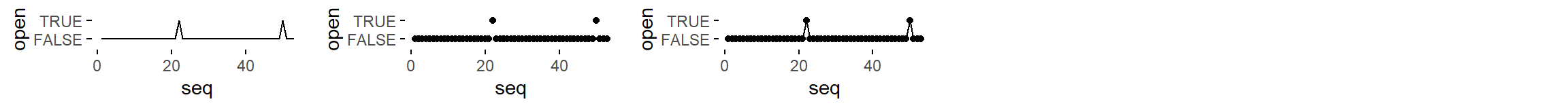``logical = c('line graph', 'point graph', 'point-to-point graph')``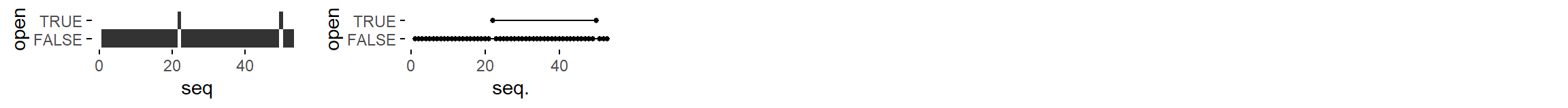``logical = c('tile plot', 'linerange graph')``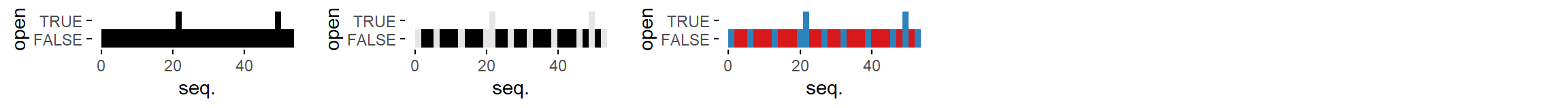``logical = c('binned heatmap', 'bw binned heatmap', 'color binned heatmap')``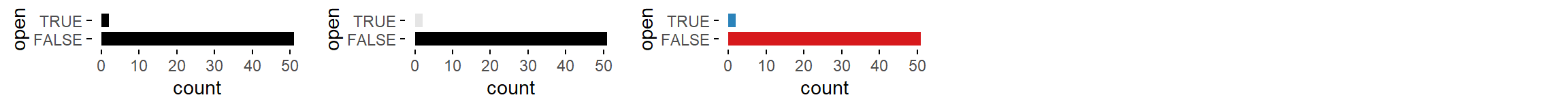``logical = c('bar graph', 'bw bar graph', 'color bar graph')``

# Ordered factor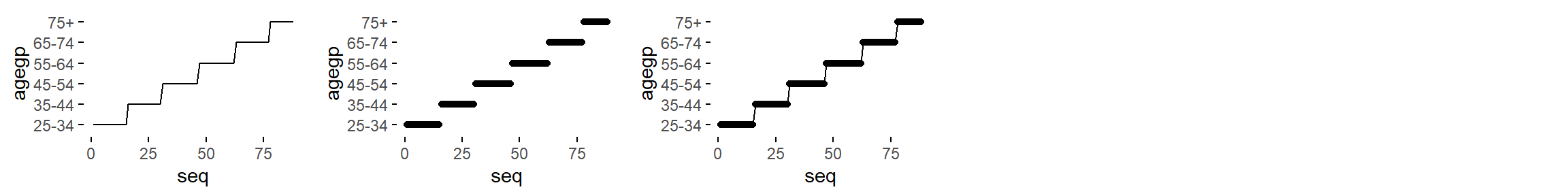``ordered = c('line graph', 'point graph', 'point-to-point graph')``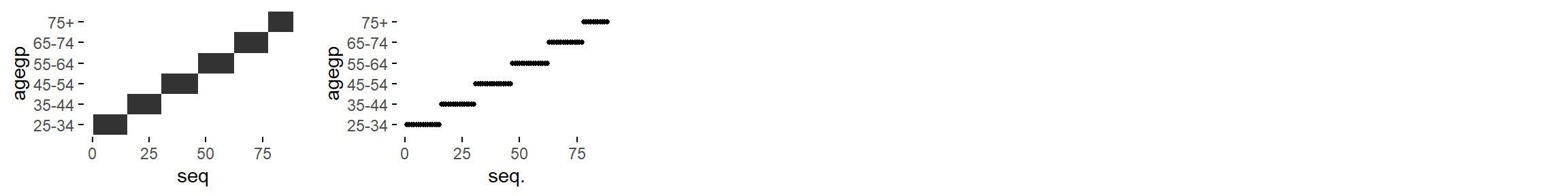``ordered = c('tile plot', 'linerange graph')``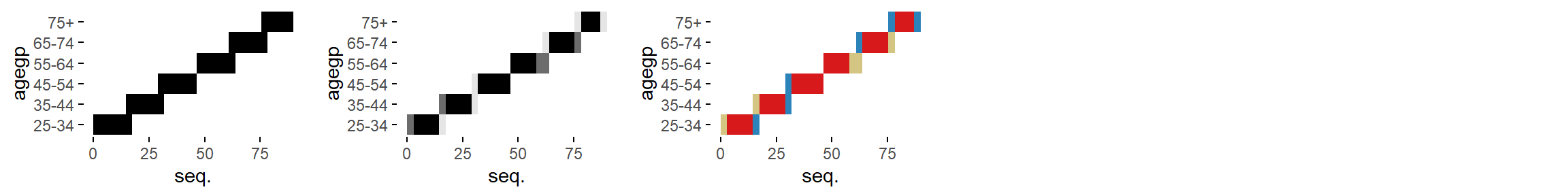``logical = c('binned heatmap', 'bw binned heatmap', 'color binned heatmap')``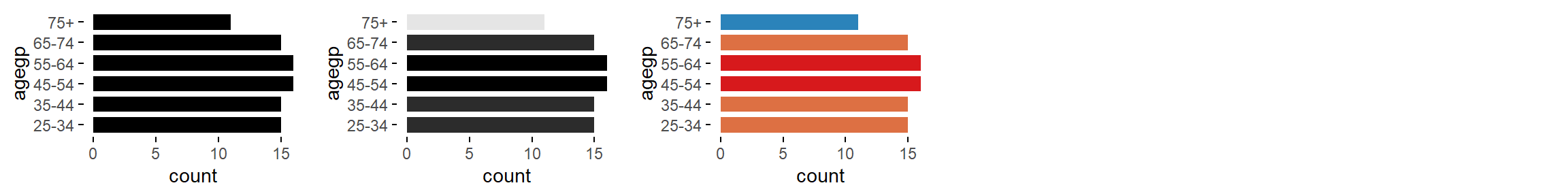``ordered = c('bar graph', 'bw bar graph', 'color bar graph')``

# Factor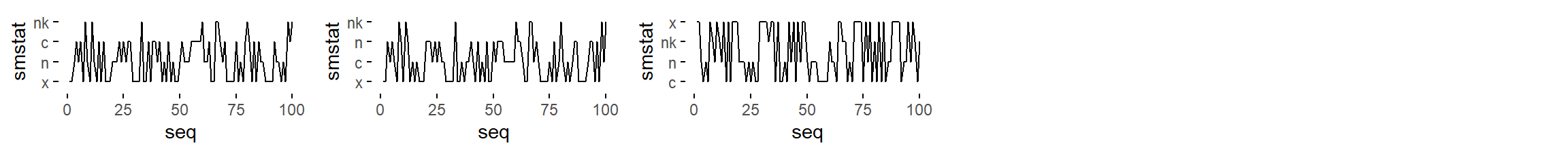``factor = c('line graph', 'freq. reordered line graph', 'alphab. reordered line graph')``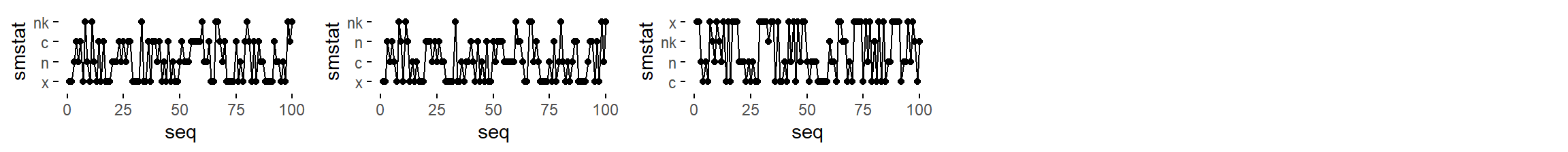``factor = c('point-to-point graph', 'freq. reordered point-to-point graph', 'alphab. reordered point-to-point graph')``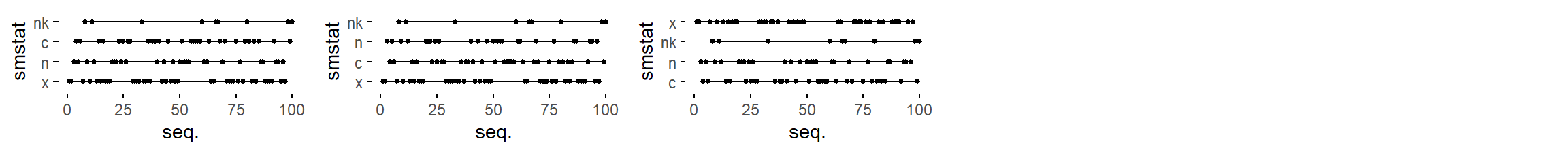``factor = c('linerange graph', 'freq. reordered linerange graph', 'alphab. reordered linerange graph')``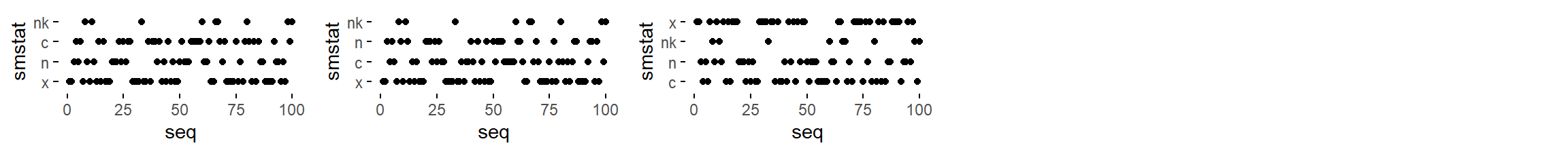``factor = c('point graph', 'freq. reordered point graph', 'alphab. reordered point graph')``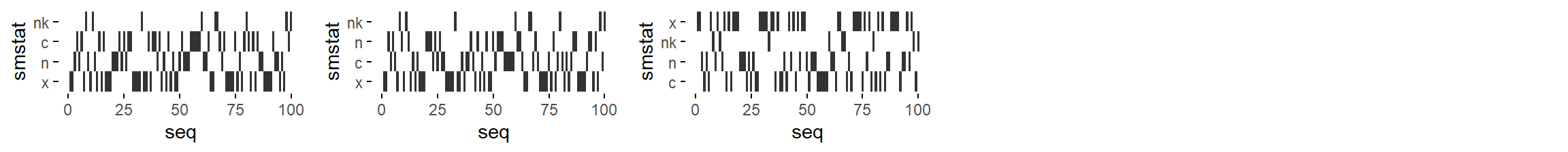``factor = c('tile plot', 'freq. reordered tile plot', 'alphab. reordered tile plot')``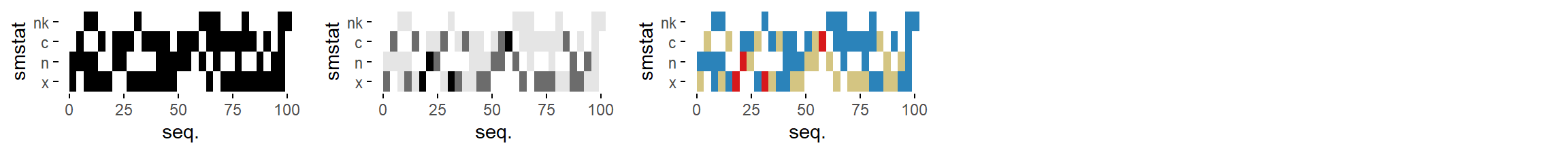``factor = c('binned heatmap', 'bw binned heatmap', 'color binned heatmap')``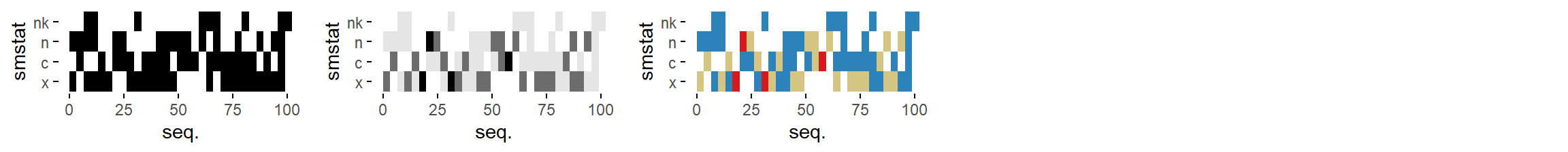``````factor = c('freq. reordered binned heatmap',
'bw freq. reordered binned heatmap',
'color freq. reordered binned heatmap')``````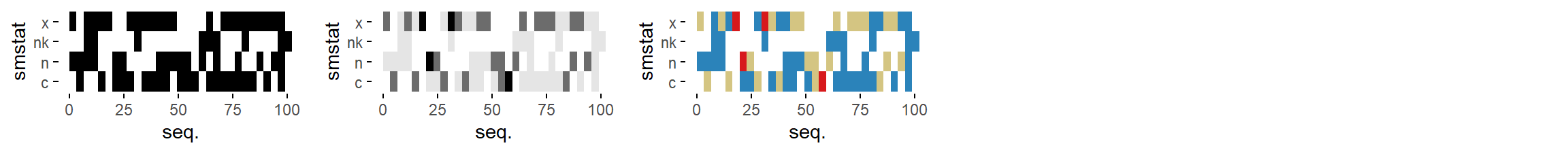``````factor = c('alphab. reordered binned heatmap',
'bw alphab. reordered binned heatmap',
'color alphab. reordered binned heatmap')``````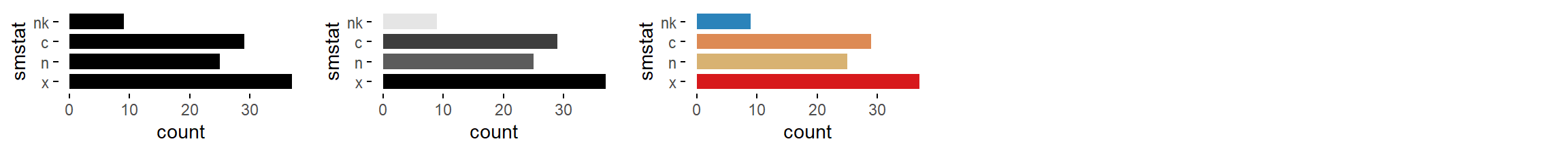``factor = c('bar graph', 'bw bar graph', 'color bar graph')``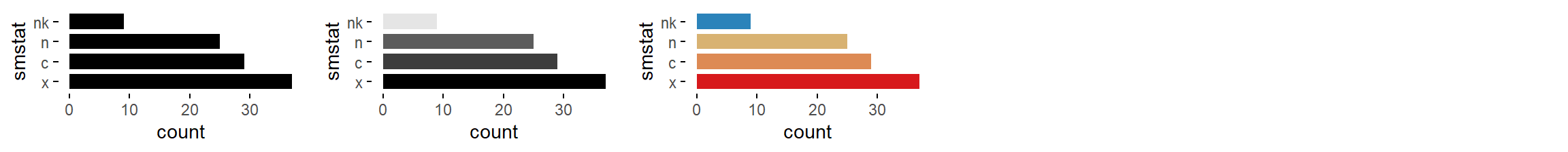``factor = c('freq. reordered bar graph', 'bw freq. reordered bar graph', 'color freq. reordered bar graph')``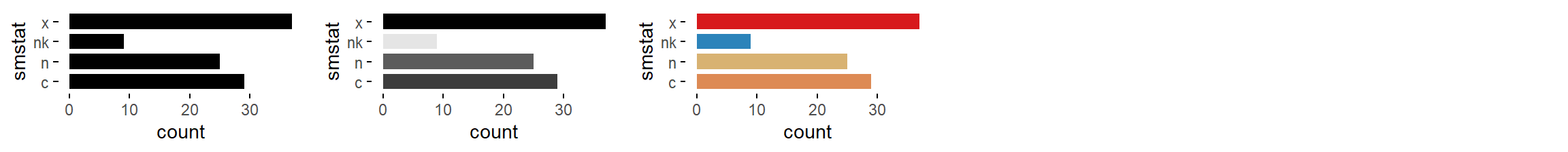``factor = c('alphab. reordered bar graph', 'bw alphab. reordered bar graph', 'color alphab. reordered bar graph')``

# Character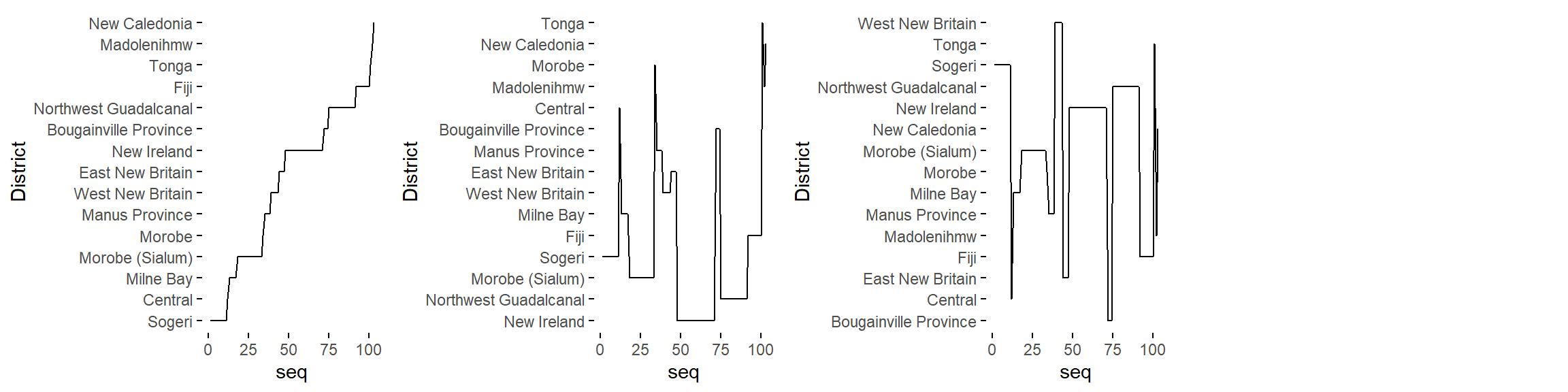``character = c('line graph', 'freq. reordered line graph', 'alphab. reordered line graph')``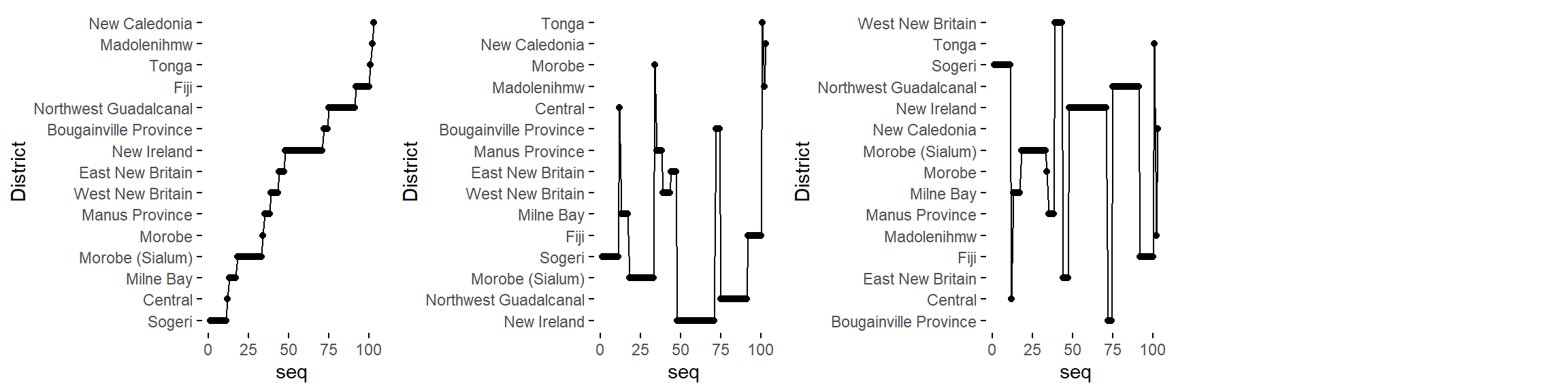``character = c('point-to-point graph', 'freq. reordered point-to-point graph', 'alphab. reordered point-to-point graph')``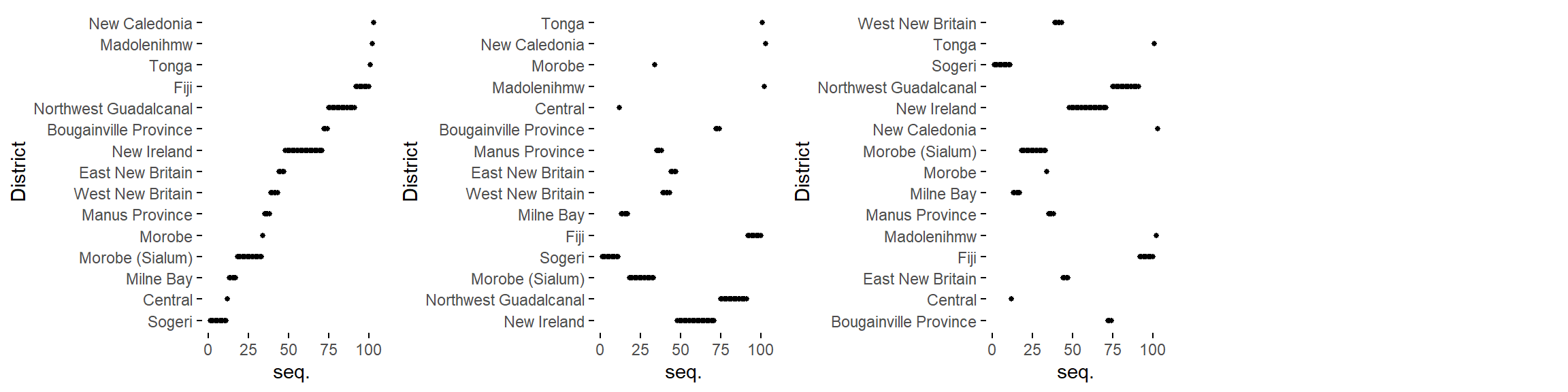``character = c('linerange graph', 'freq. reordered linerange graph', 'alphab. reordered linerange graph')``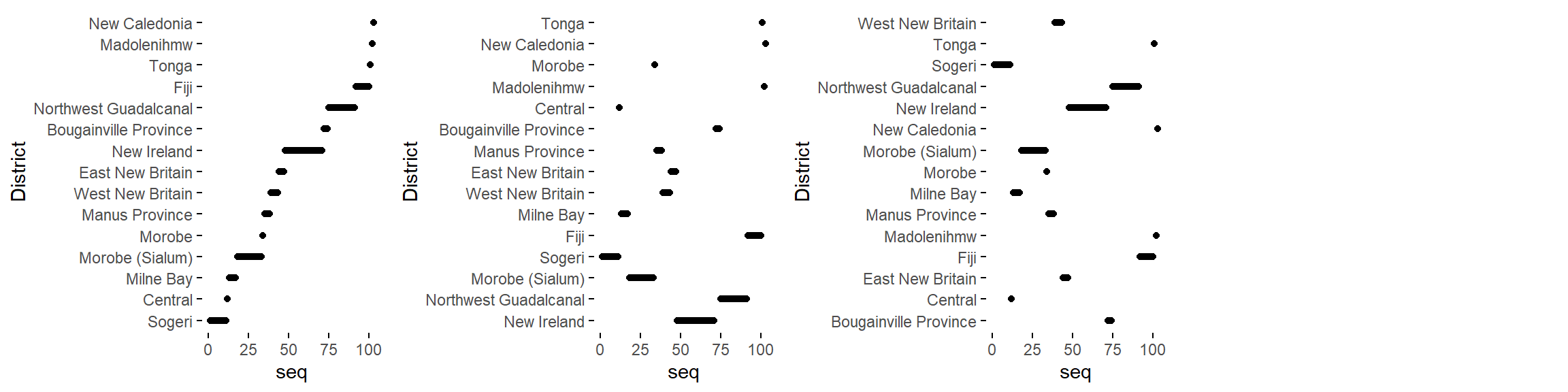``character = c('point graph', 'freq. reordered point graph', 'alphab. reordered point graph')``